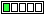All about flooble | fun stuff | Get a free chatterbox | Free JavaScript | Avatarsperplexus dot infoPolynomial and exponential (Posted on 2023-09-15)Determine all pairs of positive integers (x, y) such that x4 - 6x2 + 1 = 7·2y

 No Solution Yet Submitted by Danish Ahmed Khan No RatingComments: ( Back to comment list | You must be logged in to post comments.)Puzzle Solution Attempt| Comment 1 of 3
The lhs x^4-6x^2+1 factorises as: (x^2+2x-1)(x^2-2x-1)
Thus (x^2+2x-1)(x^2-2x-1) =7*2^y
Thus, at least one of the factors in the lhs is divisible by 7.
By trial and error, we observe that:
x^2+2x-1=0(mod 7), whenever x=2, or 3
However, x=2 gives the value of the lhs as: -7<0. This is a contradiction.
Thus, x=3, so that:
(3^2+2*3-1)(3^2-2*3-1) = 14*2=28
Thus, 7*2^y = 28,
Or, 2^y=4, giving: y=2
Therefore, (x,y) = (3,2) is the required solution to the given equation.
Note: Haven't  been able to prove that x= 2, 3 (mod7), does NOT yield further solutions.

Edited on September 15, 2023, 7:41 am
 Posted by K Sengupta on 2023-09-15 07:26:40Please log in:

 Search: Search body:
Forums (0)